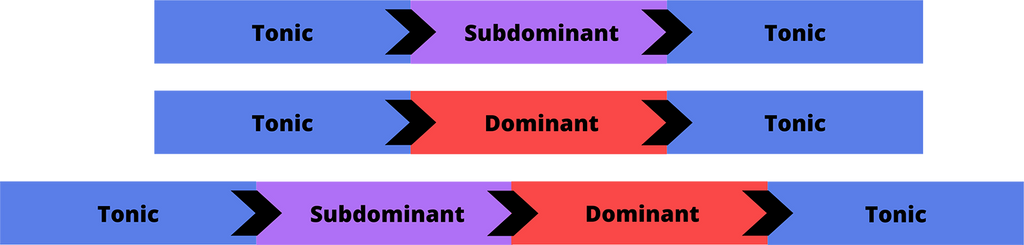# Harmonic Function in Music!

### We will learn the mechanics of harmonic function, and of course, how to use it!# So… What is Harmonic Function?

### The notes that compile a scale aren’t just thrown there, each note has its own part of the chain and will act differently, depending on its place.

In any given scale, some notes will be “stable” and others “unstable” and these notes will be compiled into chords that will also sound “stable” or “unstable”.

The “unstable” chords will always aspire to be resolved into a “stable” chord. This distance between “stable” and “unstable” can be stretched in all sorts of ways and is the key to understanding the works of harmony.

### The notes that compile the C major scale are C-D-E-F-G-A-B. In this table, we can see where every note wants to resolve.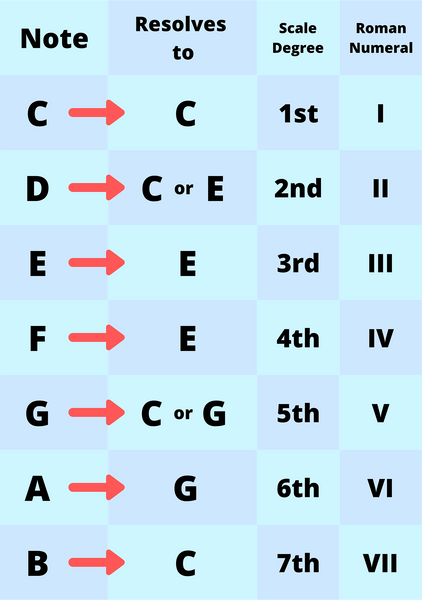### Now let’s analyze what we see:

All the notes of C major scale resolve into either C, E, or G which are the notes that compile the C major chord.

This chord, in comparison to the others, is the most stable one and gives the listener a sense of “resolution” like coming home from a trip. This feeling is the tonality of the scale!

Two notes we also need to pay attention to are the 4th and the 7th. They are the only notes located only a semitone above their neighboring note. This close distance creates a strong movement inside the scale.

### The rules of this system go like this:

• A chord that contains the 3rd degree of the scale (E in this case) and does not contain the 4th (F) will be considered as a Tonic chord.

• A chord that contains the 4th degree of the scale (F) will be considered as a subdominant

chord.

• A chord that contains the 4th (F) and 7th (B) degrees of the scale will be considered as a dominant chord.

# the Tonic Function

### These are the degrees that feel the most stable and give us a sense of resolution.

We can get this sound from a triad chord, a seventh chord, with or without tensions, a two-note interval, or even from one note alone.
Remember, it is a sense, a feeling, and not a specific note or voicing!

Now, let’s see what the Tonic chords are.
Remember, these are the chords that contain the 3rd-degree note (E) of the C major scale:

### The Tonic Chords

The 1st-degree chord, C major (C) - C-E-G

The 3rd-degree chord, E minor (Em) - E-G-B

The 6th-degree chord, A minor (Am) - A-C-E

### Within these 3 chords, there is a hierarchy, levels of stability.

The 1st degree (C major chord) is the most stable because it contains the whole C major chord notes in it, C-E-G.

The 6th degree (A minor chord) is also stable but less stable than C. It is second in the Tonic hierarchy because it still contains the C note, which is a very strong note in C major scale. In its seventh chord shape (Am7) it contains the whole C major chord triad notes, A-C-E-G.

The 3rd degree (E minor chord) is stable but last in the Tonic hierarchy. It does not contain the C note but it does contain the other notes of the C major triad chord, E-G-B.Note: musicians can refer to a note or a chord as a degree and it can mean different things. for example, when speaking about C note in the C major scale we will call it the 1st degree. When talking about degrees of a specific chord, Dm, for example, we can refer to D as the 1st degree (the 1st degree of the chord.

# The Dominant Function

The Dominant degrees are the most unstable, we will feel the highest tension ⚡️ and desire for resolution from these degrees.

Now, let’s see what the Dominant chords are. Remember, these are the chords that contain the 4th and 7th-degree notes (F and B) of the C major scale:

### The Dominant Chords

The 5th-degree chord, G major (G) - G-B-D-(F)

The seventh chord format of G in the C major scale is G7.

The 7th-degree chord, B diminished (Bo) - B-D-F-(A)

The seventh chord format of this chord is Bø (can be written like this as well - Bm7b5).

### These two chords are also different in their levels of tensity.

The G7 chord not only contains the 4th and 7th notes, but it also contains the G note as well which desires to be resolved into C (as mentioned in the table above), therefore it carries the most tension out of these two Dominant chords.

The Bø chord contains the 4th and 7th degrees but there isn’t a G in it, therefore it is a Dominant chord but carries less tension than the G7.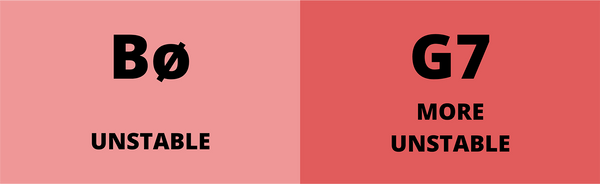# the Subdominant Function

The Subdominant degrees carry mid-level tension  ⚖️ because they contain only the 4th degree of the scale (F). They feel unresolved but not as tense as the dominant chords.

Now, let’s see what the Subdominant chords are. Remember, these are the chords that contain only the 4th-degree note (F) of the C major scale:

### The Subdominant Chords

The 2nd-degree chord, D minor (Dm) - D-F-A

The 4th-degree chord, F major (F) - F-A-C

### Within these 2 chords, there are also different levels of stability.

The 2nd degree (D minor chord) is tenser than F major because it contains the D note which carries more desire for resolution than A.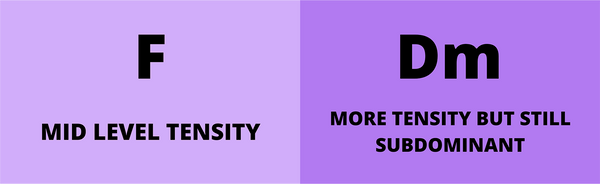### Let’s sum up everything into a table: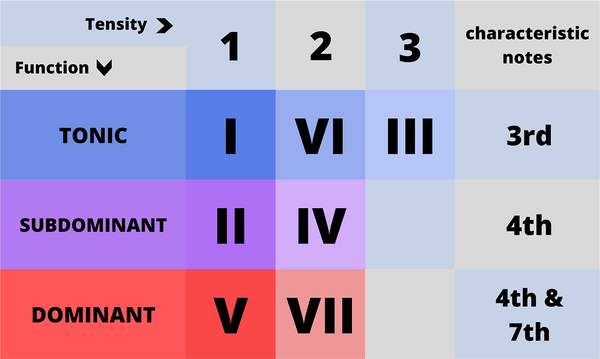### Placing an unstable chord before a more stable chord creates a sense of movement and change, let’s see how we can format harmonic movements using their harmonic function: